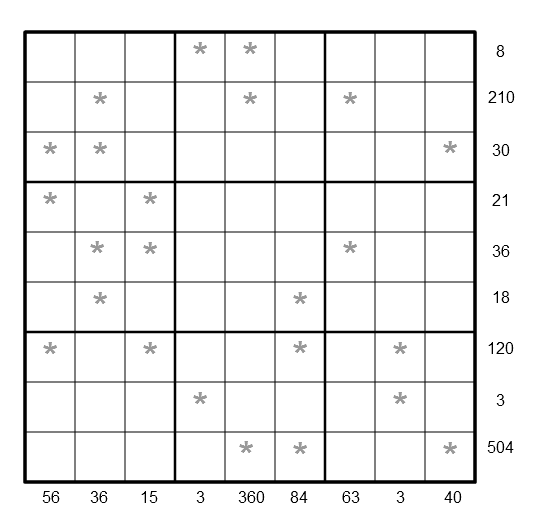#### You may also likeMake a set of numbers that use all the digits from 1 to 9, once and once only. Add them up. The result is divisible by 9. Add each of the digits in the new number. What is their sum? Now try some other possibilities for yourself!### Old Nuts

In turn 4 people throw away three nuts from a pile and hide a quarter of the remainder finally leaving a multiple of 4 nuts. How many nuts were at the start?### Some Cubes

The sum of the cubes of two numbers is 7163. What are these numbers?

# LCM Sudoku II

#### By Henry Kwok### Rules of LCM Sudoku

Like the standard sudoku, this sudoku variant has the basic rule:
• Every row, every column and every $3\times 3$ box in the grids contains the digits $1$ through $9$.
The puzzle can be solved by finding the values of the unknown digits (all indicated by asterisks) in the squares of the $9\times 9$ grid. At the bottom and right side of the $9\times 9$ grid are numbers, each of which is the Least Common Multiple (LCM) of a column or row of unknown digits marked by asterisks.

Altogether $18$ Least Common Multiples of $9$ columns and $9$ rows of unknown digits are given as clues for solving the puzzle.

As an example, the LCM of $3$, $9$, $6$, $4$, $2$ and $1$ is $36$. In the puzzle, the LCM of the four unknown digits in the second column beginning from the left of the $9\times 9$ grid is $36$, while the LCM of the four unknown digits in the seventh row beginning from the top of the $9\times 9$ grid is $120$.

After finding the values of all the unknown digits, the puzzle is solved by the usual sudoku technique and strategy.